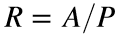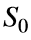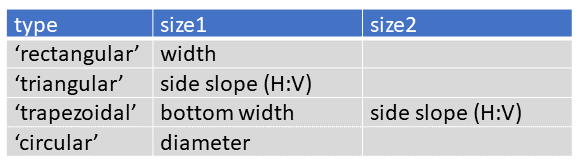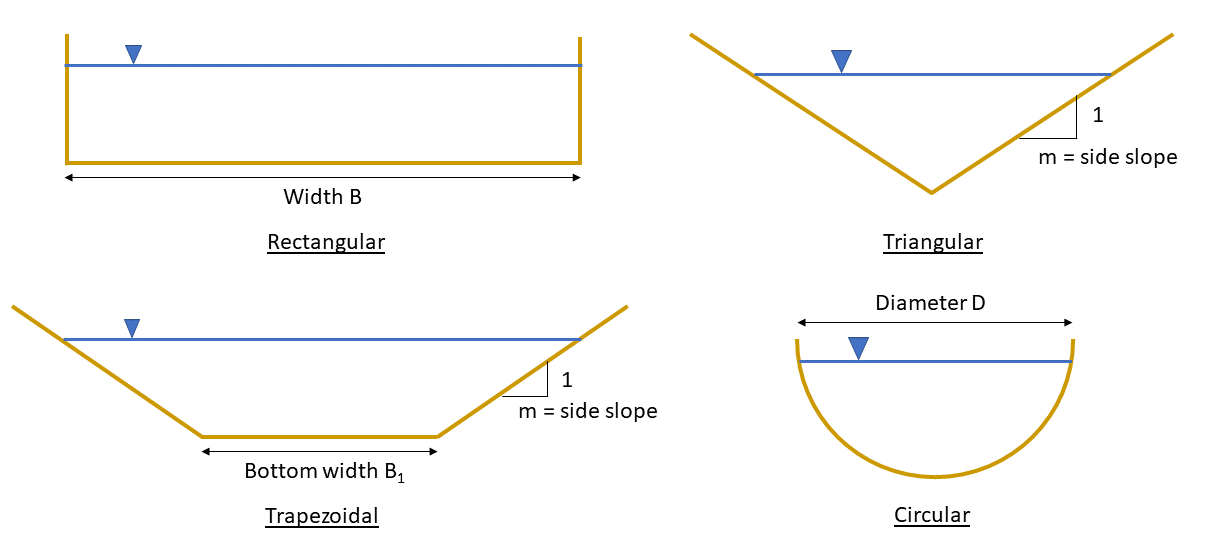# Problem 58314. Compute the normal depth of a channel

For steady uniform flow in a channel of constant cross section and slope, the depth does not change with distance or time. In this state, the component of the fluid’s weight down the slope is balanced by the friction from the wetted perimeter \$P\$, the portion of the perimeter touched by the fluid.
As described in Cody Problem 57969, the normal depth, or depth of flow in this state, can by computed from Manning’s equation for the discharge (or flow) Q):where n is Manning’s roughness coefficient,is the hydraulic radius,is the slope of the channel, and A is the cross-sectional area of the flow. The coefficient C is 1 for SI units and 1.49 for U.S. customary units.
Write a function to compute the normal depth of a channel. The unit system will be specified in units as either ‘SI’ or ‘USCS’ (U.S. customary system). The geometry of the channel’s cross section will be specified by a structure channelStruct with the following fields and possible values:The side slope is the horizontal distance divided by the vertical distance; for example, a side slope of 1.5 would mean the bank rises 1 m for every 1.5 m in the horizontal direction.### Solution Stats

75.0% Correct | 25.0% Incorrect
Last Solution submitted on Jun 14, 2023

### Community Treasure Hunt

Find the treasures in MATLAB Central and discover how the community can help you!

Start Hunting!